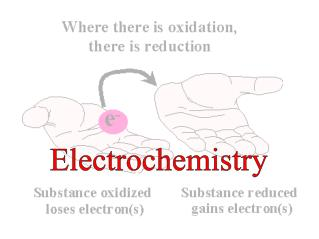DownloadDownload PresentationElectrochemistry

# Electrochemistry

Télécharger la présentation## Electrochemistry

- - - - - - - - - - - - - - - - - - - - - - - - - - - E N D - - - - - - - - - - - - - - - - - - - - - - - - - - -
##### Presentation Transcript

1. Electrochemistry

2. Define oxidation and reduction. • Determine oxidation numbers for atoms. • Identify the oxidizing agent, the reducing agent. • Distinguish between redox and non-redox reactions.

3. Burning and corrosion needs oxygen – oxidation. Oxidation-reduction reactions – (redox) Chemical changes when electrons are transferred from one reactant to another. Oxidation - an atom loses one or more electrons. Reduction- an atom gains one or more electrons. "LEO says GER”  Losing Electrons is Oxidation, Gaining Electrons is Reduction

4. 2 Mg (s) + O2 (g) → 2 MgO (s) Mg – neutral Mg2+ ion O – neutral O2– ion Magnesium is oxidized. Oxygen is reduced. O + 2e- → O2- Mg → Mg2+ + 2e-

5. Mg (s) + Cl2 (g) → MgCl2 (s) Written difference between ion and oxidation: Chlorine ion – Cl1- ion charge = 1-  oxidation number = -1 Cl + 1e- → Cl1- Mg → Mg2+ + 2e- Sometimes these numbers are the same (like above) sometimes they are very different – which is why we write them differently.

6. Oxidation number represents the chargethe atom would haveifevery bond were ionic. **You will assign them to EACH atom** 1. Assign known numbers first (below). Then calculate the others. • All uncombined elements (& diatomics) – zero. • (unless otherwise stated as charge) • Monatomic ion in ionic bonds -equals ion charge.

7. In compounds: • Alkali metals - always +1. • Earth metals - always +2 • Al: +3, F: -1, H: +1*, O: –2 Neutral compound: Sum of ox.numbers from each atom must be zero. Charged compound: Sum of ox.numbers must be charge of compound. Assign oxidation numbers to each atom in SO2. S = +4 O = –2

8. Assign ox.numbers for each atom in K2Cr2O7 Step 1: Start with atoms which are known. O: –2  K: +1 Step 2: Solve for other atoms. Neutral compound: Sum of ox.numbers from each atom must be zero. +6 +1 -2 2 K2CrO7 +2 ?? +12 = 0 -14 K = +1 Cr = +6 O = –2.

9. Assign ox. numbers for each atom in Fe(NO3)3 Step 1: Start with atoms which are known. O: –2  Fe: +3 Step 2: Solve for other atoms. Neutral compound: Sum of ox.numbers from each atom must be zero. +5 +3 -2 3 Fe(NO3) +3 +15 = 0 -18 ?? Fe = +3 N = +5 O = –2.

10. Use ox.numbers to determine if reaction is a redox reaction. -2 +1 -2 +4 +4 +1 -2 SO2 + H2O → H2SO3 +4 -2 +2 -4 +2 -6 +4 Ox.numbers do not change – no e- transferred – NOT a redox reaction.

11. Is the following reaction a redox reaction? -2 +5 0 +1 -2 +1 +5 0 Cu(s) + 2 AgNO3(aq) → CuNO3(aq) + 2 Ag(s) 0 +5 +1 -6 +5 +1 0 -6 • Cu – oxidized (loss of electrons).   • Ag – reduced (gain of electrons). Oxidation cannot occur without reduction.

12. Oxidizing agent - causes the oxidation of another substance. AgNO3 is the oxidizing agent Reducing agent - causes the reduction of another substance. Cu is the reducing agent Oxidizing agent becomes reduced and the reducing agent becomes oxidized. +1 0 Cu(s) + 2 AgNO3(aq) → CuNO3(aq) + 2 Ag(s) +1 0

13. Identify the substance oxidized, the substance reduced, the oxidizing agent and the reducing agent. +1 0 -2 +2 -2 -2 +1 +1 +5 -2 2 HNO3(aq) + 3 H2S(g) → 2 NO(g) + 3 S(s) + 4 H2O(l) +2 0 +5 +1 -2 +2 -2 +2 -6 -2 S – oxidized N – reduced H2S – reducing agent HNO3 – oxidizing agent

14. How many electrons are transferred in the reaction below: gains 3e- +1 0 -2 +2 -2 -2 +1 +1 +5 -2 3 HNO3(aq) + H2S(g) → 2 NO(g) + 3 S(s) + 4 H2O(l) 2 loses 2e- S: (3 atoms) x (2e- lost) = 6 electrons lost N: (2 atoms) x (3e- gained) = 6 electrons gained Stoichiometry used to determined total electrons transferred.

15. Strong oxidizing agents: Are very reactive – will take from anything Oxidizing Agents Reaction Products O2 O2–, H2O, CO2 F2, Cl2, Br2, I2 F–, Cl–, Br–, I– MnO4– Mn2+ Cr2O72– Cr3+ HNO3 NO, NO2 H2O2 O2, H2O Strong reducing agents: Are very reactive – will give to anything. Metals, substances that burn easily – H2, CxHy